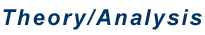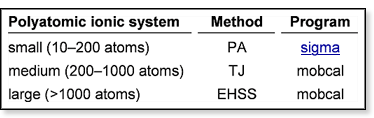Introduction     Experimental cross sections determined in an ion mobility experiment are, without doubt, dependent on ion shape. This has clearly been demonstrated, as in the case of carbon clusters. However, because experimental cross sections are the result of an ion-buffer gas scattering process, it is not straightforward to understand how the Cartesian coordinates of a theoretical candidate structure can be converted into a collision cross section. How is the collision process best be described? Is scattering elastic or inelastic? Early work on ion mobility theory lead the way, indicating it is the momentum transfer collision integral that is the quantity that should be calculated. Unfortunately, these integrals are difficult to evaluate when dealing with nonspherical systems and can only be calculated numerically. However, we have found that simple projection cross sections are - after careful calibration - in many cases, an excellent approximation to the collision integral. The guidelines in the table below represent our present understanding of which method is best used for a given system. PA denotes "projection approximation" (i.e. the shadow of a structure) and is calculated by a program developed in our group (sigma).[3,4,5] TJ denotes a "trajectory" calculation on a 12-6-4 potential (Lennard-Jones + ion-induced dipole) centered at the position of each atom  and EHSS ("exact hard sphere scattering") a trajectory calculation using hard spheres centered at the position of each atom. For TJ and EHSS the program mobcal can be obtained from Martin Jarrold's group at Indiana University.TJ works well for any size system, but calculations are very expensive. Hence, PA and EHSS calculations are preferred. However, for large systems PA fails because the details of the scattering process are obviously not taken into account. For small systems, EHSS fails because the ion-buffer gas interaction becomes important compared to the geometry of the ion and careful calibration of the atomic radii is essential. This type of calibration is implemented in our program sigma by using an adjusted 12-6-4 potential to evaluate each atomic radius within a given polyatomic ion.[4,5] For medium sized systems we don't presently know a feasible method to bypass the expensive TJ calculations. Introduction The Sigma Program References See also: "Theory/Analysis: Ion Mobility Theory"